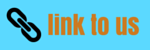Test Series-3

## Instructions

A. Question paper format:
• The question paper consists of Physics Part. There are 3 sections.
• Section I contains 7 multiple choice questions. Each question has 4 choices (A), (B), (C) and (D) for its answer, out of which only one is correct.
• Section II contains 7 multiple choice questions. Each question has 4 choices (A), (B), (C) and (D) for its answer, out of which only one is correct.
• Section III contains 1 questions. Each question has 4 choices (A), (B), (C) and (D) for its answer, out of which only one is correct.

B. Marking scheme:
• For each question in Section I you will be awarded 2 marks for the correct answer and zero mark if question is not attempted.
• For each question in Section II, you will be awarded 3 marks for the correct choice(s) for the answer, and zero mark if question is not attempted.
• For each question in Section III you will be awarded 2 marks for the correct answer and zero mark if question is not attempted.

SECTION-I

Single Correct Choice Type

This section contains 5 multiple choice questions. Each question has 4 choices (A), (B), (C) and (D) for its answer, out which ONLY ONE is correct
1.A particle of mass m = 6 kg is moving with a uniform speed v = 3 m/sec in the XOY plane along the straight line Y = X - 4 . The magnitude of the angular momentum about origin is
• 36(2)1/2
• 18(2)1/2
• 64(2)1/2
• 60

2.Five Charges of equal amount (Q) are placed at five corners of a regular hexagon of side 15 cm. What will be the value of sixth charge placed at sixth corner of the hexagon so that the electric field at the centre of hexagon is zero
• Q/6
• Q/2
• Q
• none of these

3.(N+n) number of identical resistors each of resistance R is combined to get the minimum and maximum resistances, what is the ratio of the maximum to minimum resistance
• 1:(N+n)2
• (N+n)2:1
• 1:(N+n)
• (N+n):1

4.what is true for a elastic collision?
• Both Linear momentum and Energy are conserved
• Linear momentum is conserved but Energy is not conserved
• Energy is conserved but Linear momentum is not conserved
• Both Linear momentum and Energy are not conserved

5.A massless and inextensible cord is wound round the circumference of a circular ring of mass M and radius R. The ring is free to rotate about an axis passing through its centre and perpendicular to its plane. A mass m is attached at the free end of the cord and is at rest. The angular speed of the ring when mass m has fallen through at height h is
• √(2gh/R2)
• √(2mgh/MR2)
• √[2mgh/(M + 2m)R2]
• √[2mgh/(M + m)R2]

6.A concrete sphere of radius R has a cavity of radius r which is packed with sawdust. The relative densities of concrete and sawdust are 2.4 and 0.3 respectively. For this sphere to float with its entire volume submerged under water, the ratio of the mass of concrete to the mass of sawdust will be
• 4
• 8
• 3
• None of these

7.A gas behaves more closely as an ideal gas at
• low pressure and low temperature
• low pressure and high temperature
• high pressure & low temperature
• high pressure & high temperature

SECTION-IV

Paragraph -I A solid cylinder of Mass M and Radius R rolls down an inclined plane of height H

8.Calculate the Angular velocity of the cylinder when it reaches bottom of the plane
• (4gH/3R2)1/2
• (2gH/3R2)1/2
• (gH/3R2)1/2
• (4gH/R2)1/2

9.Find the linear velocity of the cylinder when it reaches at the bottom
• (4gH/3)1/2
• (2gH/3)1/2
• (gH/3)1/2
• None of these

10.Rotational energy of the cylinder when it reaches the bottom of the plane
• MgH/4
• 2MgH/3
• MgH
• MgH/3

Paragraph -II Two metallic sphere A and B of Radii R1 and R2 are far apart.They are connected by a thin wire.
Q1 is the charge on metallic sphere A
Q2 is the charge on metallic sphere B
And it is given Q1 +Q1 =Q

11.Find the value of Q1
• R1Q/(R1+R2)
• R2Q/(R1+R2)
• R1Q/(R1-R2)
• None of these

12.Find the value of Q2
• R1Q/(R1+R2)
• R2Q/(R1+R2)
• R2Q/(R1-R2)
• none of these

13.Find the potential of Metallic sphere A
• Q/4πε0(R1+R2)
• Q/4πε0R1
• Q/4πε0R2)
• None of these

14.Find the potential of Metallic sphere B
• Q/4πε0(R1+R2)
• Q/4πε0R2
• Q/4πε0R1
• None of these

SECTION-III

Single Correct Choice Type

This section contains 1 multiple choice questions. Each question has 4 choices (A), (B), (C) and (D) for its answer, out which ONLY ONE is correct
15. Two statements are made STATEMENT-I: Two bodies of mass m and M will reach ground in same time when fallen at same time from the tower of height h
STATEMENT-II: Same Acceleration due to gravity is experienced by the both body in fall from tower
• Statement I is true ,statement II is true ,statement II is correct explanation for statement I
• Statement I is true ,statement II is true ,statement II is not a correct explanation for statement I
• Statement I is true,Statement II is false
• Statement I is False,Statement II is True